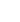15min:
HIGH RESOLUTION MICROWAVE SPECTROSCOPY OF CH AS A SEARCH FOR VARIATION OF FUNDAMENTAL CONSTANTS.

S. TRUPPE, R. J. HENDRICKS, S. K. TOKUNAGA, E. A. HINDS, M. R. TARBUTT, Centre for Cold Matter, Blackett Laboratory, Imperial College London, London, SW7 2BW.

The Standard Model of particle physics assumes that fundamental, dimensionless constants like the fine-structure constant,, or the ratio of the proton to electron mass, µ, remain constant through time and space. Laboratory experiments have set tight bounds on variations of such constants on a short time scale. Astronomical observations, however, provide vital information about possible changes on long time scales. Recent measurements using quasar absorption spectra provide some evidence for a space-time variation of the fine-structure constant. It is thus important to verify this discovery by using an entirely different method. Recently the prospect of using rotational microwave spectra of molecules as a probe of fundamental constants variation has attracted much attention. Generally these spectra depend on µ, but if fine and hyperfine structure is involved they also become sensitive to variations ofand the nuclear g-factor. Recent calculations, show that the-doublet and rotational spectra of CH are particularly sensitive to possible variations of µ and. We present recent laboratory based high-resolution spectra of the-doublet transition frequencies of the \mathcalF2, J=1/2 and \mathcalF1, J=3/2 states of CH, X2~(v=0) at 3.3GHz and 0.7GHz respectively, with \mathcalF labelling the different spin-orbit manifolds of CH. We also present a measurement of the transition frequency between the two spin-orbit manifolds \mathcalF2, J=1/2 and \mathcalF1, J=3/2 at 530GHz. By using a molecular beam of CH in combination with a laser-microwave double-resonance technique and Ramsey's method of separated oscillatory fields, we have measured these transition frequencies to unprecedented accuracy. Hence CH can now be used as a sensitive probe to detect changes in fundamental constants by comparing lab based frequencies to radio-astronomical observations from distant gas clouds.